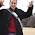## Wednesday, January 6, 2010

### Matching in for-comprehensions

At a glance a for-comprehension appears to be equivalent to a Java for-loop, but it is much much more than that. As shown in post: for-comprehensions, for-comprehensions can have guards which filter out which elements are processed:
`scala> for ( x <- 1 to 10; if (x >4) ) println(x)5678910`
They can be used to construct new collections:
`scala>?for(?i?<-?List(?"a",?"b",?"c")?)?yield?"Word:?"+ires1:?List[java.lang.String]?=?List(Word:?a,?Word:?b,?Word:?c)`
They can contain multiple generators:
`scala> for {x <- 1 to 10                                |      if(x%2 == 0)     |      y <- 1 to 5} yield (x,y)res1: scala.collection.immutable.IndexedSeq[(Int, Int)] = IndexedSeq((2,1), (2,2), (2,3), (2,4), (2,5), (4,1), (4,2), (4,3), (4,4), (4,5), (6,1), (6,2), (6,3), (6,4), (6,5), (8,1), (8,2), (8,3), (8,4), (8,5), (10,1), (10,2), (10,3), (10,4), (10,5))`
What has not been covered is that the assignments also does pattern matching:
`scala> for ( (x,y) <- (6 to 1 by -2).zipWithIndex) println (x,y) (6,0)(4,1)(2,2)`
This is not surprising as this also occurs during normal assignment. But what is interesting is that the pattern matching can act as a guard as well. See Extractor examples and Assignment and Parameter Objects for more information of pattern matching and extractors.
`scala> val args = Array( "h=2", "b=3")args: Array[java.lang.String] = Array(h=2, b=3)scala> val Property = """(.+)=(.+)""".r Property: scala.util.matching.Regex = (.+)=(.+)scala> for {Property(key,value) <- args } yield (key,value)res0: Array[(String, String)] = Array((h,2), (b,3))scala> Map(res0:_*)res1: scala.collection.immutable.Map[String,String] = Map(h -> 2, b -> 3)scala> res1("h")res3: String = 2`
Now just for fun here is a similar example but using symbols instead of strings for the key values:
`scala> val args = Array( "h=2", "b=3")args: Array[java.lang.String] = Array(h=2, b=3)scala> val Property = """(.+)=(.+)""".r Property: scala.util.matching.Regex = (.+)=(.+)scala> for {Property(key,value) <- args } yield (Symbol(key),value)res0: Array[(Symbol, String)] = Array(('h,2), ('b,3))scala> Map(res0:_*)res1: scala.collection.immutable.Map[Symbol,String] = Map('h -> 2, 'b -> 3)scala> res1('h)res2: String = 2`

1.Thank you!

I like your blog. It is really helpfull to have this little examples demonstrated and explained.

With best regards

2.I never knew about that regex syntax - quite nice. Could do do a small article on scala regex at some point?

Keep up the good work with the blog, ive learned quite a few new scala tricks here.

3.I agree with whakojacko that a Daily Scala on regex would be nice. I re-read http://stackoverflow.com/questions/1332574/common-programming-mistakes-for-scala-developers-to-avoid today and realized about pattern matching with regexes (see last answer).

It's much more convenient than the corresponding Java way of parsing Strings with regular expressions.

4.Hello Jesse, thanks for the great blog.

By coincidence I was reading Lachlan O'Dea's blog http://lachlanrambling.blogspot.com/2009/12/some-simple-scala-null-tricks.html before this article and he covered another example of matching in a for comprehension where he used an extractor to filter a generator:

for (NotNull(x) <- xs) yield x

where NotNull was defined as:

object NotNull{def unapply[A <: AnyRef](ref: A): Option[A] = if (ref eq null) None else Some(ref)}

5.That is a cool use of the for-comprehension.

2 point:

1. Scala 2.8 makes that extractor easier to write:
scala> object NotNull{def unapply[A <: AnyRef](ref: A): Option[A] = Option(ref) }
defined module NotNull

scala> for (NotNull(x) <- List(1,2,null,'c')) yield x
res0: List[AnyRef] = List(1, 2, c)

2. This is obviously a toy example because if all you wanted was to get rid of nulls using xs filter { _ != null } is must easier. But there are very good uses of combining several generators in a for-comprehension when this can be quite useful